# Wavenumber

Light, phase and group velocities (rewritten for December 2007!)

New for December 2007! In a traveling plane wave, wavenumber is the spatial equivalent of frequency. If you stopped time, the wavenumber tells you how many radians there are per unit length. Wavenumber is denoted by lower case letter "k".

In order to better understand wavenumber, we have to start with the Helmholtz equation, which can be derived from Maxwell's Equations if you're a better man that the rest of us (and you're welcome to take over this part of the lesson!) The Helmholtz equation is a partial differential equation: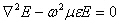For a plane wave of angular frequency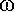traveling in the x direction, the solution to the Helmholtz equation is of the form: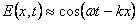Where k is the wavenumber, which is given by: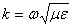Electromagnetic waves in any bulk material move at the velocity of light "vlight" that is a function of permeability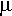and permittivity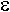of the material: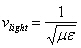Note that many textbooks call the velocity of light in a material the "phase velocity", but we're trying to reserve that term for something else. The velocity of light in free space is denoted by lower-case letter "c" and is defined as a electromagnetic plane wave's velocity in a vacuum (where the relative permeability and relative permittivity are both unity):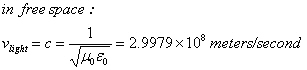The velocity of light in a bulk material can be expressed as the velocity of light in free space divided by the square-roots of the relative permeability and relative permittivity of the material. The term "velocity factor" is often refres to this material property: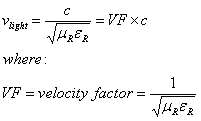Wavenumber can be expressed as function of a material's relative permeability and permittivity, along with frequency and velocity, or along with wavelength: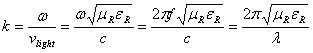In free space the relative permeability and relative permittivity are both unity, and the velocity of the wave is the same as simply the speed of light in vacuum: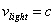The free-space wavenumber can be expressed as a function of frequency and velocity, or just the wavelength: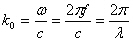Wavenumber is often confused with the propagation phase constant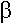. We make the following distinction (and hopefully some smarter people will agree). The phase constant refers to transmission lines, while the wavenumber refers to a plane wave. Note that for TEM transmission lines such as coax and stripline, the wavenumber and the propagation phase constant are indeed equal, but in non-TEM media such as waveguide they can be very different!

Author : Unknown Editor